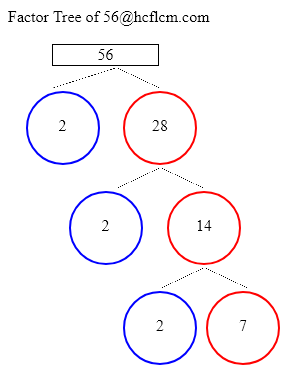# Factors of 56 | Find the Factors of 56 by Factoring Calculator

Factoring Calculator calculates the factors and factor pairs of positive integers.

Factors of 56 can be calculated quickly with the help of Factoring Calculator i.e. 1, 2, 4, 7, 8, 14, 28, 56 positive integers that divide 56 without a remainder.

Factors of 56 are 1, 2, 4, 7, 8, 14, 28, 56. There are 8 integers that are factors of 56. The biggest factor of 56 is 56.

Factors of:

### Factor Tree of 56 to Calculate the Factors

 56 2 28 2 14 2 7Factors of 56 are 1, 2, 4, 7, 8, 14, 28, 56. There are 8 integers that are factors of 56. The biggest factor of 56 is 56.

Positive integers that divides 56 without a remainder are listed below.

• 1
• 2
• 4
• 7
• 8
• 14
• 28
• 56

• 1 × 56 = 56
• 2 × 28 = 56
• 4 × 14 = 56
• 7 × 8 = 56
• 8 × 7 = 56
• 14 × 4 = 56
• 28 × 2 = 56
• 56 × 1 = 56

### Factors of 56 Table

FactorFactor Number
1one
2two
4four
7seven
8eight
14fourteen
28twenty eight
56fifty six

### How to find Factors of 56?

As we know factors of 56 are all the numbers that can exactly divide the number 56 simply divide 56 by all the numbers up to 56 to see the ones that result in zero remainders. Numbers that divide without remainder are factors and in this case below are the factors

1, 2, 4, 7, 8, 14, 28, 56 are the factors and all of them can exactly divide number 56.

### Frequently Asked Questions on Factors of 56

1. What are the factors of 56?

Answer: Factors of 56 are the numbers that leave a remainder zero. The ones that can divide 56 exactly i.e. factors are 1, 2, 4, 7, 8, 14, 28, 56.

2.What are Factor Pairs of 56?